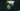# Binary Tree Data Structure

August 17, 2020A Binary tree is a data structure which has two children nodes attached to it, called left and right node. Do remember, its different from a Binary Search tree.

Few Basics if Binary Tree:

• Parent node can have maximum two children nodes
• Parent node can have Zero nodes as well, it will be the leaf node
• There is no relation in the data between parent and children.

## Basic Data Structure

Lets look at the basic data structure to denote a Binary Tree.

``````public class Node {
public int data;
public Node left;
public Node right;

public Node(int data) {
this.data = data;
this.left = null;
this.right = null;
}
}``````

Above code is in Java. A tree node has three things:

1. The data (It can be any data type, I have taken integer for simplicity)
2. A pointer/reference to left child
3. A pointer/reference to right child

Note the data type is same for left and right node.

Lets take a look at a representation of a Binary Tree:

``````        50
/    \
80      30
/    \      \
20     40      10``````

## Create Sample Binary Tree with code

Lets see a small code on how we can create above tree with the `class Node` data structure.

``````public Node buildSampleTree() {
Node root = new Node(50);
root.left = new Node(80);
root.right = new Node(30);

root.left.left = new Node(20);
root.left.right = new Node(40);

root.right.right = new Node(10);

return root;
}``````

In above sample function, we are creating our tree with the data I shown above. For `Node 30`, we have only right child. Its left pointer/reference is null. Similarly for all leaf nodes, their left and right child are null.

## Some Considerations

This data structure can be used at any place where you want to represent upto 2 children. But there is no relationship among the node values.

For example, To search an element you need to search entire tree. Since you can not determine whether your node lies in left or right side, unlike Binary Search tree